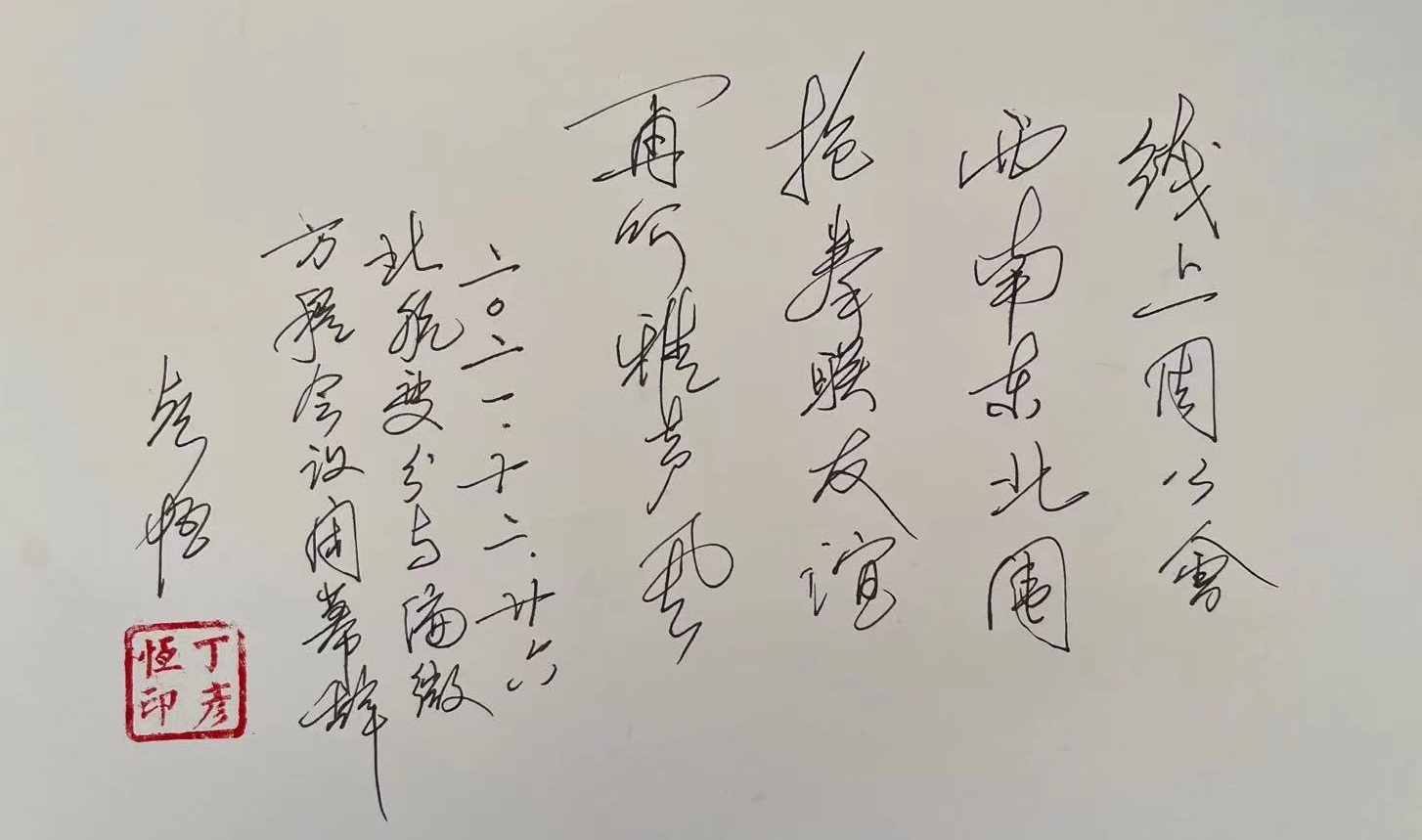# Calculus of Variations and PDE

## 相关信息

 丁彦恒 中科院数学研究所 冯伟杰 北京航空航天大学 蒋美跃 北京大学 阮元龙 北京航空航天大学(2021-12-25)

～～～～～～～

## 12月25日议程

(报告为随机排序)

08:35-09:00 Dec. 25

09:00-09:40 Dec. 25
Transition between nonlinear and linear eigenvalue problems

We study convergence of variational solutions of the nonlinear eigenvalue problem $$-\Delta u=\lambda|u|^{p-2}u, u\in H_0^1(\Omega),$$ as $p\downarrow2$ or as $p\uparrow2$, where $\Omega$ is a bounded domain in $\mathbb R^N$ with smooth boundary. It turns out that if $\lambda$ is not an eigenvalue of $-\Delta$ then the solutions either blow up or vanish according to $p\downarrow2$ or $p\uparrow2$, while if $\lambda$ is an eigenvalue of $-\Delta$ then the solutions converge to the associated eigenspace.

09:45-10:25 Dec. 25
Non-degeneracy of Multi-bubbling Solutions for fractional prescribed curvature problem

We consider the following fractional prescribed scalar curvature problem $$\left\{\begin{array}{ll} (-\Delta)^s u =K(y)u^{2^*_s-1}, \ \hbox{ in } \ \mathbb{R}^N, \\ u>0, \quad u \in \dot{H}^s(\mathbb{R}^N), \end{array}\right.$$ where $N > 2s+2$, $\frac{1}{2} < s <1$, $2^*_s=\frac{2N}{N-2s}$ is the critical Sobolev exponent, $\dot{H}^s(\mathbb{R}^N)$ is the completion of $C_0^{\infty}(\mathbb{R}^N)$ under the semi-norm $[u]_{H^s(\mathbb{R}^N)} :=(\int_{\mathbb{R}^N}|(-\Delta)^{\frac{s}{2}}u|^2dx)^{\frac{1}{2}}$, and $K(y)$ is a positive radial function. We first prove a non-degeneracy result for the positive bubbling solutions of the equation via the local Pohozaev identities. Then we apply the non-degeneracy result to construct new bubbling solutions by Lyapunv-Schmidt reduction arguments. It should be mentioned that due to the non-localness of the fractional operator, we can not establish the local Pohozaev identities for the solution of $u$ directly. Instead, we consider its corresponding harmonic extension, and this difference not only makes the sharp estimates for several integrals in the local Pohozaev identities but also force us to use the Pohozaev identities in quite a different way.

10:25-10:35 Dec. 25

10:35-11:15 Dec. 25
Bifurcation of nodal solutions of the mean curvature space-like equation in certain Friedmann-Lemaitre-Robertson-Walker space times

We study the global bifurcation of nodal solutions of the mean curvature spacelike equation in certain Friedmann-Lemaitre-Robertson-Walker space times: $\left( \frac{\nabla u}{f\left( u\right) \sqrt{f\left(u\right) ^{2}-\left\vert \nabla u\right\vert ^{2}}}\right)$ $+\frac{f^{\prime}\left( u\right) }{\sqrt{f\left( u\right)^{2}-\left\vert \nabla u\right\vert ^{2}}}\left( n+\frac{\left\vert \nabla u\right\vert ^{2} }{f\left( u\right) ^{2}}\right)$ $=nH\left( u,\left\vert x\right\vert \right) \text{ in }B\left( R\right)$, $\left\vert \nabla u\right\vert <f\left( u\right) \text{ in }B\left( R\right)$ and $\frac{\partial u}{\partial\nu} =0 \text{ on }\partial B\left( R\right).$

11:20-12:00 Dec. 25
Small Solitons and Multi-Solitons in Nonlinear Schrödinger Equations

By solving two types of constrained variational problems and spectrum analysis, we find an approach to ascertain frequency from the prescribed mass for nonlinear Schrödinger equations. Then we prove existence and orbital stability of small solitons depending on frequencies for nonlinear Schrödinger equation with competitive power nonlinearity. In addition, we construct multi-solitons of the equation with different speeds by stable solitons.

12:00-14:00 Dec. 25

14:00-14:40 Dec. 25
Heisenberg群上的分析或非线性退化椭圆方程及其应用

14:45-15:25 Dec. 25

15:25-15:35 Dec. 25

15:35-16:15 Dec. 25
Maslov-type index theory and periodic orbits

In this talk, I will introduce two kinds of periodic orbit problems, including closed geodesics on compact manifolds and closed characteristics on hypersurfaces in $R^{2n}$. And I will also focus on explaining how the Maslov-type index theory has been applied to do with these corresponding problems.

16:20-17:00 Dec. 25

18:00-00:00  Dec. 25

## 12月26日议程

(报告为随机排序)

08:30-09:10 Dec. 26

09:15-09:55 Dec. 26
From KP-I lump solution to traveling waves of Gross-Pitaevskii Equation

We are interested in the existence of traveling wave solutions of the GP equation. In this talk, we will use the lump solution of the KP-I equation to construct a family of traveling wave solutions of the GP equation with transonic speed. Compared with the variational method, our construction has the advantage that the precise asymptotic behavior of the solutions can be described.

10:00-10:40 Dec. 26
Local linking and its applications to indefinite Schrodinger type

In the last two decades, nonlinear Schrodinger type equations, such as Schrodinger-Poisson systems, Schrodinger-Kirchhoff equations and quasilinear Schrodinger equations, have captured great interests in the field on nonlinear analysis. Most of the results require that the Schrodinger operator is positive definite, so that the zero function is a local minimizer of the variational functional and the mountain pass theorem applies. We are interested in the case that the Schrodinger operator is indefinite. It turns out that unlike semilinear Schrodinger equations, the classical minimax theorems such as the linking theorem, are also not applicable. We found that the concept of local linking introduced by Shujie Li and Jiaquan Liu in the 1980's, is suitable for this kind of problems. In this talk I will present our recent results for such problems, obtained by applying local linking theory and Morse theory.

10:45-11:25 Dec. 26
On the scalar curvature problem with very degenerate prescribed functions

We will talk about a scalar curvature problem. It is known that there are a number of results related to the existence of solutions concentrating at the isolated critical points of the scalar curvature $K(x)$. However, if $K(x)$ has non-isolated critical points with different degenerate rate along different directions, whether there exist solutions concentrating at these points is still an open problem. We give a certain positive answer to this problem via applying a blow-up argument based on local Pohozaev identities and modified finite dimensional reduction method when the dimension of critical point set of $K(x)$ ranges from $1$ to $N-1$.(2021-12-26)

～～～～～～～